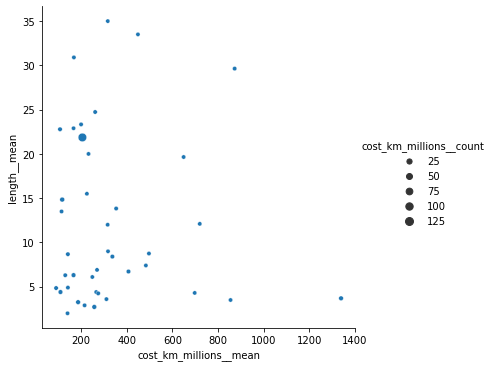Important: This post is hard to read. Experimenting with generating a notebook and posting it without a lot of extra work. Might try recording creation of a post sometime. But for now, this post is hard to parse as a user experience, although it does have an Altair graph you can poke and prod at the end...

• From TidyTuesday, read in the data and get to a visualization with Seaborn and Altair
• Quite rough, thinking it might be beneficial to try recording making a notebook...

```import pandas as pd

transit_cost = pd.read_csv('https://raw.githubusercontent.com/rfordatascience/tidytuesday/master/data/2021/2021-01-05/transit_cost.csv')
```

```transit_cost.isnull().sum()
```
```e                    0
country              0
city                 0
line                 0
start_year          46
end_year            64
rr                   1
length               0
tunnel_per          25
tunnel              26
stations             8
source1              5
cost                 0
currency             0
year                 0
ppp_rate             2
real_cost            0
cost_km_millions     2
source2              7
reference           12
dtype: int64```
```transit_cost = transit_cost[~transit_cost.e.isnull()]
```

## For the tracks that are completed, what is the relationship between cost and distance?

```transit_cost.tunnel_per.value_counts().head()
```
```100.00%    293
0.00%       60
84.00%       3
35.00%       3
53.00%       3
Name: tunnel_per, dtype: int64```
• most of the tunnels are completed
```completed = transit_cost[
(transit_cost.tunnel_per == '100.00%') &
(~transit_cost.cost_km_millions.isnull())
]
```
```completed.isnull().sum()
```
```e                    0
country              0
city                 0
line                 0
start_year          26
end_year            40
rr                   0
length               0
tunnel_per           0
tunnel               0
stations             2
source1              0
cost                 0
currency             0
year                 0
ppp_rate             0
real_cost            0
cost_km_millions     0
source2              0
reference            2
dtype: int64```
```# mean and counts for cost_km_millions by country
completed.groupby('country')['cost_km_millions'].agg(['mean', 'count']).sort_values('mean', ascending=False).head(10)
```
mean count
country
US 1339.014950 11
SG 872.836620 3
NZ 854.760857 1
UK 719.891667 2
EG 697.674419 1
PH 649.141985 2
AU 497.160784 2
HU 483.762162 1
IN 448.955224 1
CA 407.403943 6
```compare = completed.groupby('country').agg(
{
'cost_km_millions': ['mean', 'count'],
'length': ['mean']
}
)

# rename the multiIndex
compare.columns = ['__'.join(col).strip() for col in compare.columns.values]
compare.reset_index(inplace=True)
```
```compare.head()
```
country cost_km_millions__mean cost_km_millions__count length__mean
0 AR 232.300000 1 20.00
1 AT 317.777778 1 9.00
2 AU 497.160784 2 8.75
3 BE 265.909091 1 4.40
4 BG 90.285327 4 4.85

## Seaborn

```import seaborn as sns

sns.relplot(
data=compare,
x='cost_km_millions__mean',
y='length__mean',
size='cost_km_millions__count'
)
```

`<seaborn.axisgrid.FacetGrid at 0x7fb4ec86cfa0>`## Altair

```import altair as alt

alt.Chart(compare).mark_circle(size=50).encode(
x=alt.X('cost_km_millions__mean', axis=alt.Axis(title='Average Cost per Kilometer (\$Million/km)')),
y=alt.Y('length__mean', axis=alt.Axis(title='Average Length (km)')),
tooltip=['country', 'cost_km_millions__mean', 'length__mean'],
).properties(
title='Dig Dug: Building Tunnels'
).configure_mark(
opacity=0.7
).configure_title(
fontSize=24

).interactive()
```